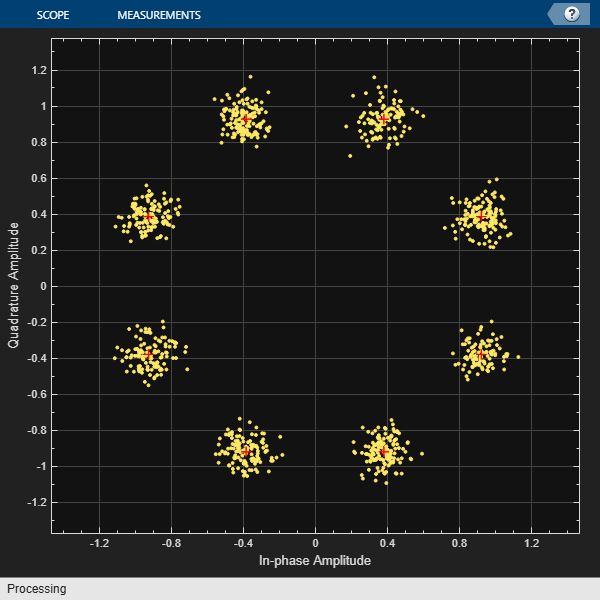Documentation

Adjust Carrier Synchronizer Damping Factor to Correct Frequency Offset

Attempt to correct for a frequency offset using the carrier synchronizer object. Increase the damping factor of the synchronizer and determine if the offset was corrected.

Set the modulation order, sample rate, frequency offset, and signal-to-noise ratio parameters.

M = 8;
fs = 1e6;
foffset = 1000;
snrdb = 20;

Create a phase frequency offset object to introduce a frequency offset to a modulated signal. Create a constellation diagram object.

pfo = comm.PhaseFrequencyOffset('SampleRate',fs, ...
'FrequencyOffset',foffset);
constDiagram = comm.ConstellationDiagram('ReferenceConstellation',pskmod(0:M-1,M,pi/M));

Create a carrier synchronizer object to correct for the frequency offset.

carriersync = comm.CarrierSynchronizer('Modulation','8PSK', ...
'DampingFactor',0.05,'NormalizedLoopBandwidth',0.01);

The main processing loop includes these steps:

• Generate random data.

• Apply 8-PSK modulation.

• Introduce a frequency offset.

• Pass the signal through an AWGN channel.

• Correct for the frequency offset.

• Display the constellation diagram.

for k = 1:200
data = randi([0 M-1],1000,1);
modSig = pskmod(data,M);
txSig = pfo(modSig);
rxSig = awgn(txSig,snrdb);
syncOut = carriersync(rxSig);
constDiagram(syncOut)
endThe constellation points cannot be clearly identified indicating that the carrier synchronizer is unable to compensate for the frequency offset.

Determine the normalized pull-in range, the maximum frequency lock delay, and the maximum phase lock delay by using the info function.

syncInfo = info(carriersync)
syncInfo =

struct with fields:

NormalizedPullInRange: 0.0044
MaxFrequencyLockDelay: 78.9568
MaxPhaseLockDelay: 130

Convert the normalised pull-in range from radians to cycles. Compare the normalized frequency offset to the pull-in range.

[foffset/fs syncInfo.NormalizedPullInRange/(2*pi)]
ans =

1.0e-03 *

1.0000    0.7071

The offset is greater than the pull-in range. This is reason that the carrier synchronizer failed to correct the frequency offset.

Change the damping factor of the synchronizer to 0.707.

carriersync.DampingFactor = 0.707;

Repeat the main processing loop.

for k = 1:200
data = randi([0 M-1],1000,1);
modSig = pskmod(data,M);
txSig = pfo(modSig);
rxSig = awgn(txSig,snrdb);
syncOut = carriersync(rxSig);
constDiagram(syncOut)
endThere are now eight observable clusters, which shows that the frequency offset was corrected.

Determine the new pull-in range. The normalized offset is less than the pull-in range. This explains why the carrier synchronizer was able to correct the offset.

syncInfo = info(carriersync);
[foffset/fs syncInfo.NormalizedPullInRange/(2*pi)]
ans =

0.0010    0.0100